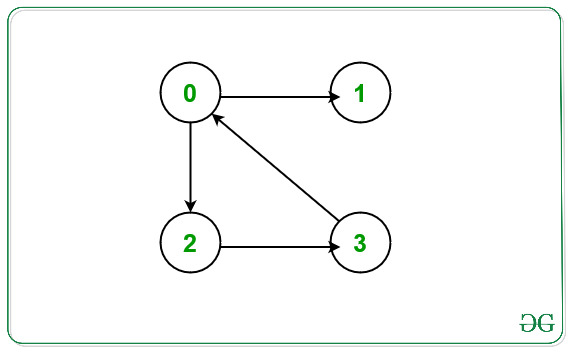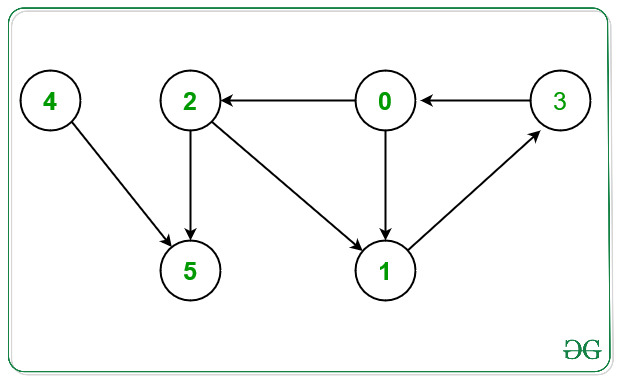# Print Nodes which are not part of any cycle in a Directed Graph

Given a directed graph G N nodes and E Edges consisting of nodes valued [0, N – 1] and a 2D array Edges[] of type {u, v} that denotes a directed edge between vertices u and v. The task is to find the nodes that are not part of any cycle in the given graph G.

Examples:

Input: N = 4, E = 4, Edges[] = { {0, 2}, {0, 1}, {2, 3}, {3, 0} }
Output: 1
Explanation:From the given graph above there exists a cycle between the nodes 0 -> 2 -> 3 -> 0.
Node which doesn’t occurs in any cycle is 1.
Hence, print 1.

Input: N = 6, E = 7, Edges[] = { {0, 1}, {0, 2}, {1, 3}, {2, 1}, {2, 5}, {3, 0}, {4, 5}}
Output: 4 5
Explanation:From the given graph above there exists a cycle between the nodes:
1) 0 -> 1 -> 3 -> 0.
2) 0 -> 2 -> 1 -> 3 -> 0.
Nodes which doesn’t occurs in any cycle are 4 and 5.
Hence, print 4 and 5.

## Recommended: Please try your approach on {IDE} first, before moving on to the solution.

Naive Approach: The simplest approach is to detect a cycle in a directed graph for each node in the given graph and print only those nodes that are not a part of any cycle in the given graph.
Time Complexity: O(V * (V + E)), where V is the number of vertices and E is the number of edges.
Auxiliary Space: O(V)

Efficient Approach: To optimize the above approach, the idea is to store the intermediate node as a visited cycle node whenever any cycle in the given graph. To implement this part use an auxiliary array cyclePart[] that will store the intermediate cycle node while performing the DFS Traversal. Below are the steps:

• Initialize an auxiliary array cyclePart[] of size N, such that if cyclePart[i] = 0, then ith node doesn’t exist in any cycle.
• Initialize an auxiliary array recStack[] of size N, such that it will store the visited node in the recursion stack by marking that node as true.
• Perform the DFS Traversal on the given graph for each unvisited node and do the following:
• Now find a cycle in the given graph, whenever a cycle is found, mark the node in cyclePart[] as true as this node is a part of the cycle.
• If any node is visited in the recursive call and is recStack[node] is also true then that node is a part of the cycle then mark that node as true.
• After performing the DFS Traversal, traverse the array cyclePart[] and print all those nodes that are marked as false as these nodes are not the part of any cycle.

Below is the implementation of the above approach:

## C++

 `// C++ program for the above approach ` ` `  `#include ` `using` `namespace` `std; ` ` `  `class` `Graph { ` ` `  `    ``// No. of vertices ` `    ``int` `V; ` ` `  `    ``// Stores the Adjacency List ` `    ``list<``int``>* adj; ` `    ``bool` `printNodesNotInCycleUtil( ` `        ``int` `v, ``bool` `visited[], ``bool``* rs, ` `        ``bool``* cyclePart); ` ` `  `public``: ` `    ``// Constructor ` `    ``Graph(``int` `V); ` ` `  `    ``// Member Functions ` `    ``void` `addEdge(``int` `v, ``int` `w); ` `    ``void` `printNodesNotInCycle(); ` `}; ` ` `  `// Function to initialize the graph ` `Graph::Graph(``int` `V) ` `{ ` `    ``this``->V = V; ` `    ``adj = ``new` `list<``int``>[V]; ` `} ` ` `  `// Function that adds directed edges ` `// between node v with node w ` `void` `Graph::addEdge(``int` `v, ``int` `w) ` `{ ` `    ``adj[v].push_back(w); ` `} ` ` `  `// Function to perform DFS Traversal ` `// and return true if current node v ` `// formes cycle ` `bool` `Graph::printNodesNotInCycleUtil( ` `    ``int` `v, ``bool` `visited[], ` `    ``bool``* recStack, ``bool``* cyclePart) ` `{ ` ` `  `    ``// If node v is unvisited ` `    ``if` `(visited[v] == ``false``) { ` ` `  `        ``// Mark the current node as ` `        ``// visited and part of ` `        ``// recursion stack ` `        ``visited[v] = ``true``; ` `        ``recStack[v] = ``true``; ` ` `  `        ``// Traverse the Adjacency ` `        ``// List of current node v ` `        ``for` `(``auto``& child : adj[v]) { ` ` `  `            ``// If child node is unvisited ` `            ``if` `(!visited[child] ` `                ``&& printNodesNotInCycleUtil( ` `                       ``child, visited, ` `                       ``recStack, cyclePart)) { ` ` `  `                ``// If child node is a part ` `                ``// of cycle node ` `                ``cyclePart[child] = 1; ` `                ``return` `true``; ` `            ``} ` ` `  `            ``// If child node is visited ` `            ``else` `if` `(recStack[child]) { ` `                ``cyclePart[child] = 1; ` `                ``return` `true``; ` `            ``} ` `        ``} ` `    ``} ` ` `  `    ``// Remove vertex from recursion stack ` `    ``recStack[v] = ``false``; ` `    ``return` `false``; ` `} ` ` `  `// Function that print the nodes for ` `// the given directed graph that are ` `// not present in any cycle ` `void` `Graph::printNodesNotInCycle() ` `{ ` ` `  `    ``// Stores the visited node ` `    ``bool``* visited = ``new` `bool``[V]; ` ` `  `    ``// Stores nodes in recursion stack ` `    ``bool``* recStack = ``new` `bool``[V]; ` ` `  `    ``// Stores the nodes that are ` `    ``// part of any cycle ` `    ``bool``* cyclePart = ``new` `bool``[V]; ` ` `  `    ``for` `(``int` `i = 0; i < V; i++) { ` `        ``visited[i] = ``false``; ` `        ``recStack[i] = ``false``; ` `        ``cyclePart[i] = ``false``; ` `    ``} ` ` `  `    ``// Traverse each node ` `    ``for` `(``int` `i = 0; i < V; i++) { ` ` `  `        ``// If current node is unvisited ` `        ``if` `(!visited[i]) { ` ` `  `            ``// Perform DFS Traversal ` `            ``if` `(printNodesNotInCycleUtil( ` `                    ``i, visited, recStack, ` `                    ``cyclePart)) { ` ` `  `                ``// Mark as cycle node ` `                ``// if it return true ` `                ``cyclePart[i] = 1; ` `            ``} ` `        ``} ` `    ``} ` ` `  `    ``// Traverse the cyclePart[] ` `    ``for` `(``int` `i = 0; i < V; i++) { ` ` `  `        ``// If node i is not a part ` `        ``// of any cycle ` `        ``if` `(cyclePart[i] == 0) { ` `            ``cout << i << ``" "``; ` `        ``} ` `    ``} ` `} ` ` `  `// Function that print the nodes for ` `// the given directed graph that are ` `// not present in any cycle ` `void` `solve(``int` `N, ``int` `E, ` `           ``int` `Edges[]) ` `{ ` ` `  `    ``// Initialize the graph g ` `    ``Graph g(N); ` ` `  `    ``// Create a directed Graph ` `    ``for` `(``int` `i = 0; i < E; i++) { ` `        ``g.addEdge(Edges[i], ` `                  ``Edges[i]); ` `    ``} ` ` `  `    ``// Function Call ` `    ``g.printNodesNotInCycle(); ` `} ` ` `  `// Driver Code ` `int` `main() ` `{ ` `    ``// Given Number of nodes ` `    ``int` `N = 6; ` ` `  `    ``// Given Edges ` `    ``int` `E = 7; ` ` `  `    ``int` `Edges[] = { { 0, 1 }, { 0, 2 },  ` `                       ``{ 1, 3 }, { 2, 1 },  ` `                       ``{ 2, 5 }, { 3, 0 },  ` `                                 ``{ 4, 5 } }; ` ` `  `    ``// Function Call ` `    ``solve(N, E, Edges); ` ` `  `    ``return` `0; ` `} `

Output:

```4 5
```

Time Complexity: O(V + E)
Space Complexity: O(V)

Attention reader! Don’t stop learning now. Get hold of all the important DSA concepts with the DSA Self Paced Course at a student-friendly price and become industry ready.

My Personal Notes arrow_drop_upCheck out this Author's contributed articles.

If you like GeeksforGeeks and would like to contribute, you can also write an article using contribute.geeksforgeeks.org or mail your article to contribute@geeksforgeeks.org. See your article appearing on the GeeksforGeeks main page and help other Geeks.

Please Improve this article if you find anything incorrect by clicking on the "Improve Article" button below.